# Cool Math Worksheet - Lumos Educational App Store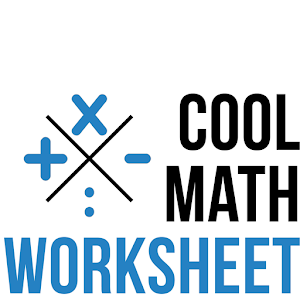41
Price -free
\$0

#### DESCRIPTION:

Cool Math Worksheets is a cool math games also can be a math workout for your kids. This math worksheet can be an addicting games. This free math game is designed for kids who wants to solve mathematics problems more quick than others. This will improve your kids so that they can do a fast math in school. Inspired by Kumon mathematics module, this coolmath game contains multiplication, division, addition, and subtraction.Using this free math worksheets as your daily math workout and . Divided into 4 level of problems : Basic Skill, Eazy Level, Medium Level, and Hard Level. This math worksheets

#### OVERVIEW:

Cool Math Worksheet is a free educational mobile app By DRXL.It helps students in grades 3,4 practice the following standards 3.OA.3,3.OA.4,3.OA.5.

This page not only allows students and teachers download Cool Math Worksheet but also find engaging Sample Questions, Videos, Pins, Worksheets, Books related to the following topics.

1. 3.OA.3 : Use multiplication and division within 100 to solve word problems in situations involving equal groups, arrays, and measurement quantities, e.g., by using drawings and equations with a symbol for the unknown number to represent the problem. .

2. 3.OA.4 : Determine the unknown whole number in a multiplication or division equation relating three whole numbers. For example, determine the unknown number that makes the equation true in each of the equations 8 .

3. 3.OA.5 : Apply properties of operations as strategies to multiply and divide. Examples: If 6 .

3
4

#### STANDARDS:

3.OA.3
3.OA.4
3.OA.5
3.OA.6
4.OA.4
4.OA.5
4.OA.A.1
4.OA.A.2
4.OA.A.3
4.OA.B.4
4.OA.C.5

Developer: DRXL

Software Version: 2.2

Category: education

Release Date: September 13, 2015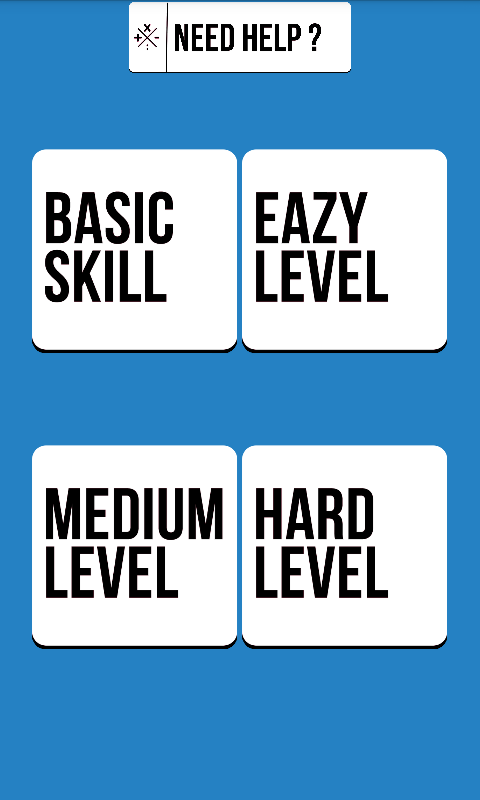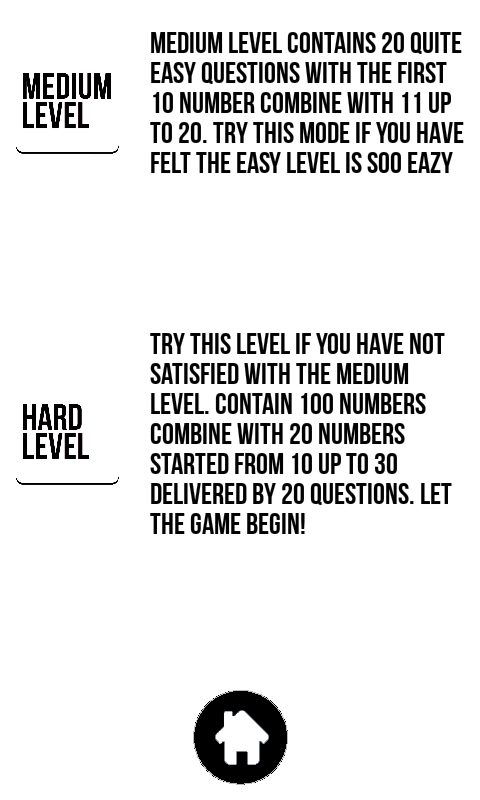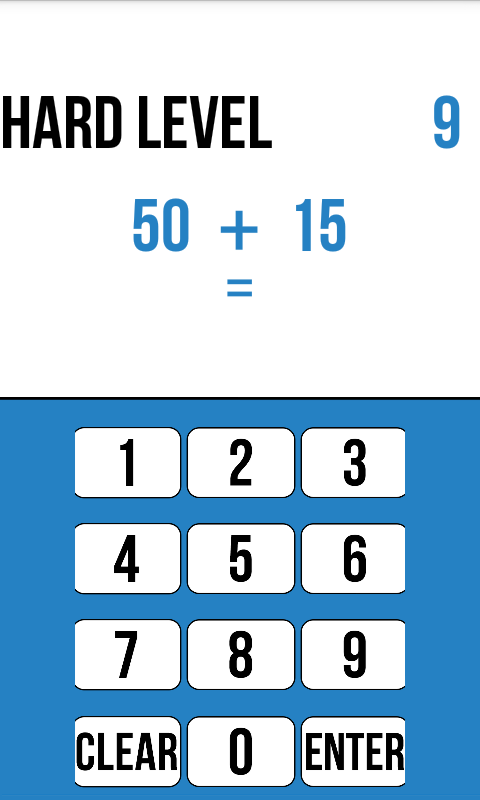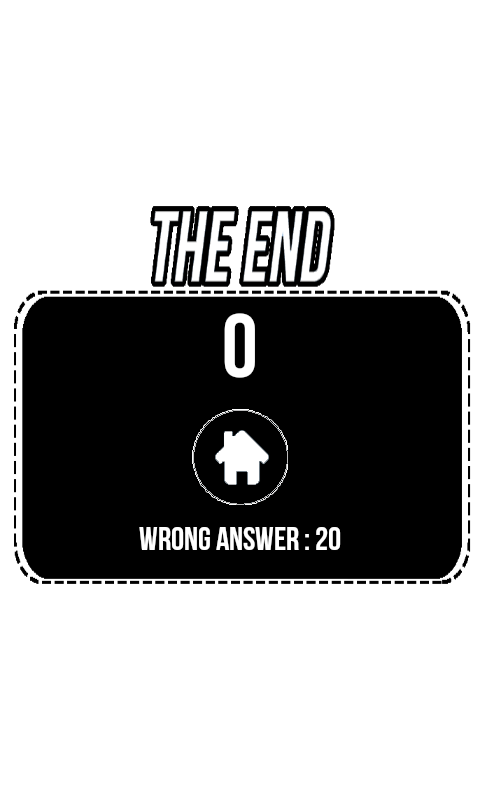### RELATED APPSEdSearch WebSearch